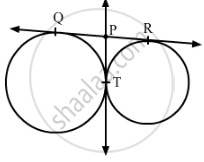# QR is a common tangent to the given circles, touching externally at the point T. The tangent at T meets QR at P. - Mathematics

In Fig. 1, QR is a common tangent to the given circles, touching externally at the point T. The tangent at T meets QR at P. If PT = 3.8 cm, then the length of QR (in cm) is :(A) 3.8
(B) 7.6
(C) 5.7
(D) 1.9

#### SolutionIt is known that the length of the tangents drawn from an external point to a circle are  equal.

∴ QP = PT = 3.8 cm               ...(1)

PR = PT = 3.8 cm                 ...(2)

From equations (1) and (2), we get:

QP = PR = 3.8 cm

Now, QR = QP + PR
= 3.8 cm + 3.8 cm
= 7.6 cm

Hence, the correct option is B.

Concept: Concept of Circle - Centre, Radius, Diameter, Arc, Sector, Chord, Segment, Semicircle, Circumference, Interior and Exterior, Concentric Circles
Is there an error in this question or solution?
2013-2014 (March) Delhi Set 1
Share